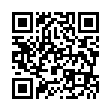# Sheet 2 2015 .pdf

### File information

Author: Dr Ashraf

This PDF 1.5 document has been generated by MicrosoftÂ® Word 2013, and has been sent on pdf-archive.com on 05/11/2015 at 22:47, from IP address 41.176.x.x. The current document download page has been viewed 1041 times.
File size: 431.3 KB (7 pages).
Privacy: public file

### Document preview

Computer Software 2015

Exercises 2.1
(1) To declare an int variable x with initial value 200, you write
a. int x = 200L;
b. int x = 200l;
c. int x = 200;
d. int x = 200.0;
(2) To declare a constant PI, you write
a. final static PI = 3.14159;
b. final float PI = 3.14159;
c. static double PI = 3.14159;
d. final double PI = 3.14159;
(3) Which of the following assignment statements is illegal?
a. float f = -34;
b. int t = 23;
c. short s = 10;
d. float f = 34.0;
(4) The assignment operator in Java is __________.
a. :=
b. =
c. = =
d. &lt;(5) Which of these data types requires the least amount of memory?
a. float
b. double
c. short
d. byte
(6) An int variable can hold __________.
a. 'x'
b. 93
c. 98.3
d. true
e. a and b

Exercise 2-2
(1) To add a to b and store result in b, you write (Note: Java is case-sensitive)
a. b += a;
b. a = b + a;
c. b = A + b;
d. a += b;

Sheet-2

Computer Software 2015

Sheet-2

(2) To assign a double variable d to an int variable x, you write
a. x = (long)d
b. x = (int)d;
c. x = d;
d. x = (float)d;
(3) Which of the following is the correct expression of character a?
a. 'a'
b. "a"
c. '\000a'
d. None of the above.
(4) Which of the following is a constant, according to Java naming conventions?
a. MAX_VALUE
b. Test
(5) An int variable can hold __________.
a. 'x'
b. 93
c. 98.3
d. true
e. a and b
(6) Which of the following assignment statements is correct to assign character 5 to c?
a. char c = '5';
b. char c = 5;
c. char c = "5";
d. char c = "344";
(7) If you attempt to add an int, a byte, a long, and a double, the result will be a __________ value.
a. byte
b. int;
c. long;
d. double;
(8) Find and correct errors in the following code:
public class Test {
public void Main(String[] args) {
int j = i + 1;
int k =5.5;
System.out.println("j is " + j + "and
k is " + k);
}
}

Exercises
(1) Translate the following steps into Java code:
Step1: Declare a double variable named miles with initial value 100;
Step2: Declare a double constant named MILE_TO_KILOMETER with value 1.609;
Step3: Declare a double variable named kilometer, multiply miles and MILE_TO_KILOMETER and
assign the result to kilometer.
Step4: Display kilometer to the console.

Computer Software 2015

Sheet-2

What is kilometer after step4?
(2) What are the benefits of using constants? Declare an int constant SIZE with value 20.
(3) Assume that int a = 1 and double d = 1.0, and that each expression is independent. What are the results of
the following expressions?
a = 46/9;
a = 46%9 + 4*4 – 2;
a = 45 + 43%5 * (23*3 % 2);
a %= 3/a + 3;
d = 4 + d*d + 4;
d += 1.5*3 + (++a);
d -= 1.5*3 + a++;
(4) Show the result of the following remainders.
56%6, 78%-4, -34%5, -34%-5, 5%1, 1%5.
(5) Arrange the following data types from largest to smallest with respect to the amount of memory required:
byte, short, int, long, float, and double.
(6) What is the result of 4/25? How would you rewrite the expression if you wished the result to be floatingpoint number?
(7) Are the following statement correct? If so, show the output.
System.out.println(“The output for 25/4 is “ + 25/4);
System.out.println(“The output for 25/4.0 is “ + 25/4.0);
(8) How would you write the following expression in Java?

4
3  d (2  a)
 9(a  bc) 
3(r  34)
a  bd
(9) Identify and fix the errors in the following code:
public class Test {
public void main(string[] args) {
int i;
int k + 100.0;
int j = i + 1;
System.out.println(“j is “ + j+ “ and k is “ +k);
}}
(10) State whether each of the following is true or false. If false, explain why.

Computer Software 2015

Sheet-2

a) Java operators are evaluated from left to right.
b) The following are all valid variable names: _under_bar_, m928134, t5, j7, her_sales\$,
his_\$account_total, a, b\$, c, z and z2.
c) A valid Java arithmetic expression with no parentheses is evaluated from left to right.
d) The following are all invalid variable names: 3g, 87, 67h2, h22, and 2h.
(11) What does an explicit conversion from a double to an int do with the fractional part of the double value?
Does casting change the variable being cast?
(12) Show the output of the following code:
public class Test {
public static void main(String[] args) {
int x1, x2, i, j, k, y, z;
float f;
x1 = 1;
x2 = 1;
y = 5 + x1--;
z = 5 + ++x2;
i = 6 % 4;
j = 1;
j += j + 3;
k = 25 / 2;
f = (float)((2 / 5) * k);

System.out.println("x1 is " + x1);
System.out.println("x2 is " + x2);
System.out.println("i is " + i);
System.out.println("j is " + j);
System.out.println("k is " + k);
System.out.println("y is " + y);
System.out.println("z is " + z);
System.out.println("f is " + f);
}
}

(13) Write declarations, statements or comments that accomplish each of the following tasks:
a) State that a program will calculate the product of three integers.
b) Declare the variables x, y, z and result to be of type int.
c) Declare the variables xVal, yVal, and zVal to be of type String.
d) Prompt the user to enter the first value, read the value from the user and store it in the variable xVal.

Computer Software 2015

Sheet-2

e) Prompt the user to enter the second value, read the value from the user and store it in the variable
yVal.
f) Prompt the user to enter the third value, read the value from the user and store it in the variable zVal.
g) Convert xVal to an int, and store the result in the variable x.
h) Convert yVal to an int, and store the result in the variable y.
i) Convert zVal to an int, and store the result in the variable z.
j) Compute the product of the three integers contained in variables x, y, and z, and assign the result to
the variable result.
k) Display a dialog containing the message "The product is " followed by the value of the variable
result.
l) Terminate the program and indicate successful termination.
(14) Using the statements you wrote in Exercise (6), write a complete program that calculates and prints the
product of three integers.
(15) What displays in the message dialog when each of the given Java statements is performed? Assume that
x = 2 and y = 3.
a) JOptionPane.showMessageDialog( null, "x = " + x);
b) JOptionPane.showMessageDialog( null, " the value of x + x is " + (x + x) );
c) JOptionPane.showMessageDialog( null, "x =" );
d) JOptionPane.showMessageDialog( null, ( x + y ) + " = " + ( y + x ) );
(16) State the order of evaluation of the operators in each of the following Java statements, and show the
value of x after each statement is performed:
a) x = 7 + 3 * 6 / 2 -1;
b) x = 2 % 2 + 2 * 2 – 2 / 2;
c) x = ( 3 * 9 * ( 3 + ( 9 * 3 / ( 3 ) ) ) );
(17) Execute the following expression:
1 + "Welcome " + 1 + 1
1 + "Welcome " + ('\u0001' + 1)
1 + "Welcome " + 'a' + 1
(18) Show and explain the output of the following code:
int 1 = 0;
System.out.println(--i + i + i++);
System.out.println(i + ++i);
(19) Which of the following are correct literals for floating-point numbers?
a. 12.3;

Computer Software 2015

Sheet-2

b. 12.3e+2;
c. 23.4e-2
d. -334.4
(20) Which of the following are correct literals for characters?
a. '1';
b. \u345d;
c. '\u3fFa';
d. '\b'

(21) Write a program that displays "Welcome to Java!" in large block letters, each letter made up of the same
character it represents. The letters should be six printed line each. For example, W is displayed as
follows:
w

w
w

w

w

w

w

w

w w

w

w w

w w

w

w

(22) Write an application that asks the user to enter two numbers, obtains the numbers from the user and
displays the larger number followed by the words "is larger" in an information message dialog. If the
numbers are equal, print the massage "These numbers are equal."
(23) Write an application that inputs from the user the radius of a circle as an integer and prints the circle's
diameter, circumference and area. Use the value 3.14159 for π.
(24) Write an application that reads an integer and determines and prints whether it is odd or even.
(25) What does the following code print?
System.out.println( "*\n**\n***\n****\n*****");
(26) Write a complete program named Problem1.java. The program reads a double number and checks
whether the number is between 1 and 1000. For example, if your input is 5, the output should be:

Is the number 5 between 1 and 1000? true
If your input is 2000, the output should be:
Is the number 2000 between 1 and 1000? False
(27) Computing the volume of a cylinder; Write a program that reads in the radius and length of a
cylinder and computes the volume using the following formulas:
volume = area * length
(28) Summing the digits of an integer: Write a program that reads an integer between 0 and 1000
and adds all the digits in the integer. For example, if an integer is 932, the sum of all digits is
14.

Computer Software 2015
Sheet-2
(29) Converting an uppercase letter to lowercase: Write a program that converts an uppercase letter
to a lowercase letter. The character is typed in the source code as a literal value.
(30) Calculating the future investment value: Write a program that reads in investment amount,
annual interest rate, and number of years, and display the future investment value using the
following formula:
futureInvestmentValue = investmentAmount x (1 + monthlyInterest Rate)numberOfYears*12

For example, if you entered amount 1000, annual interest rate 3.25%, and number of years 1, the
future investment value is 1032.98.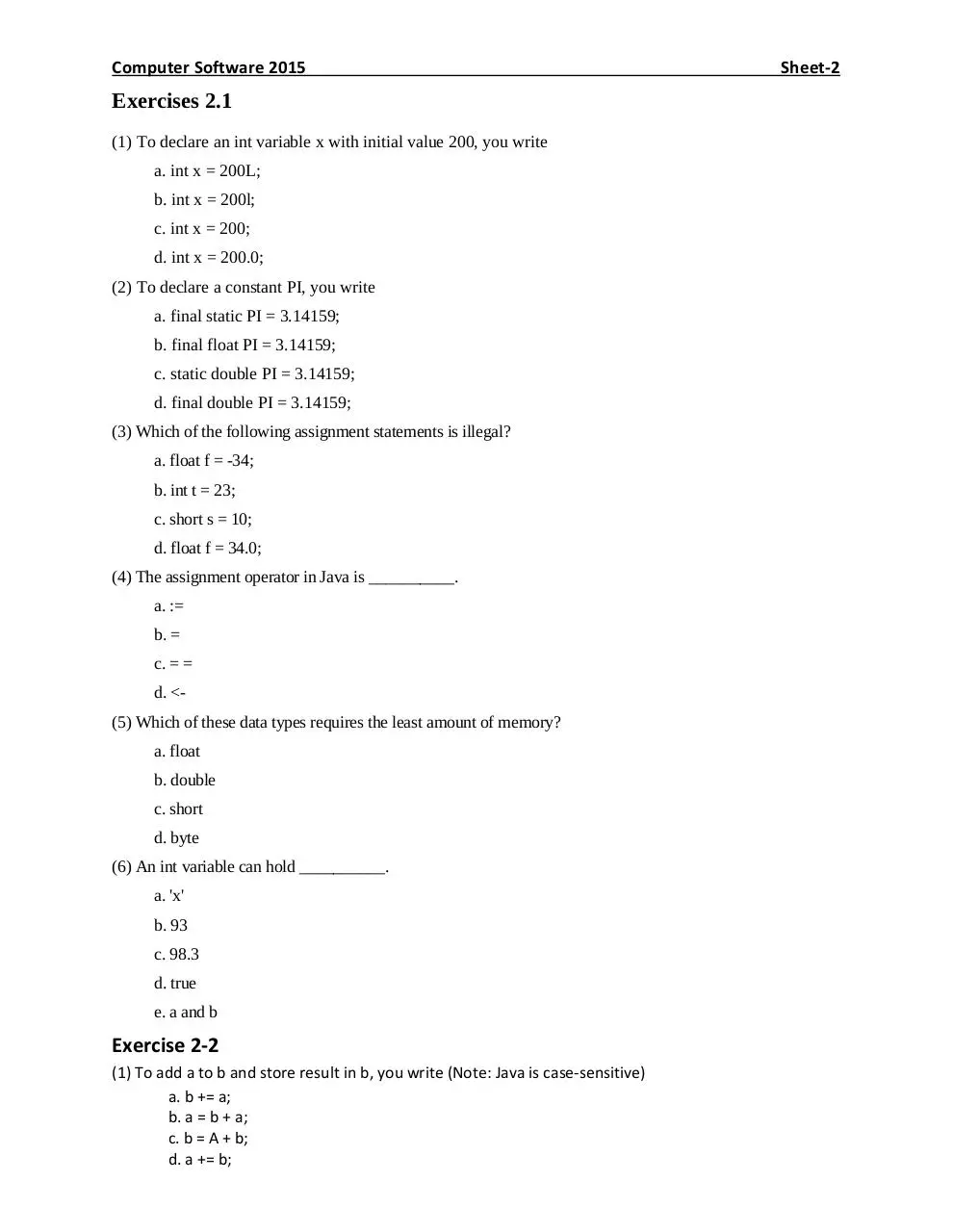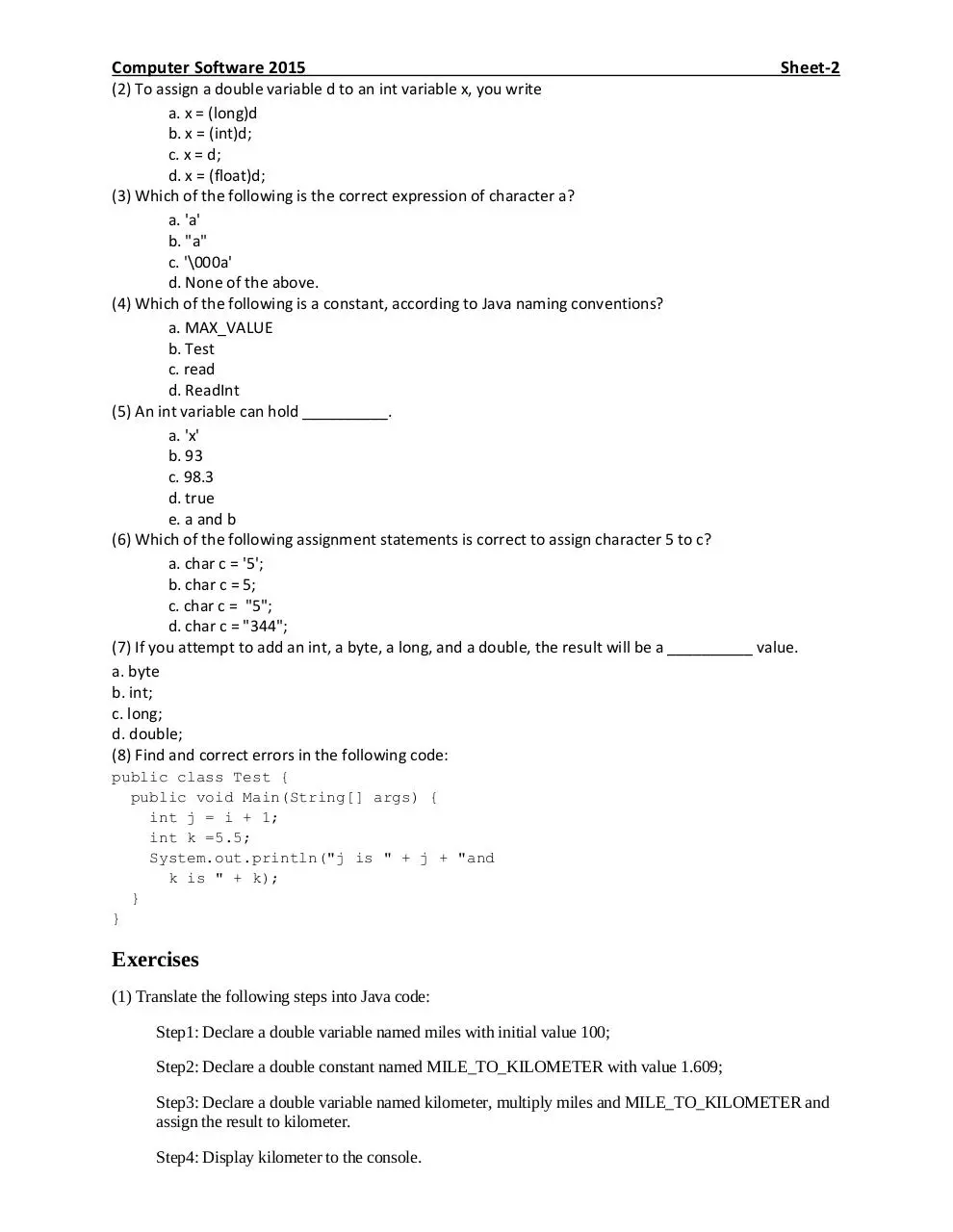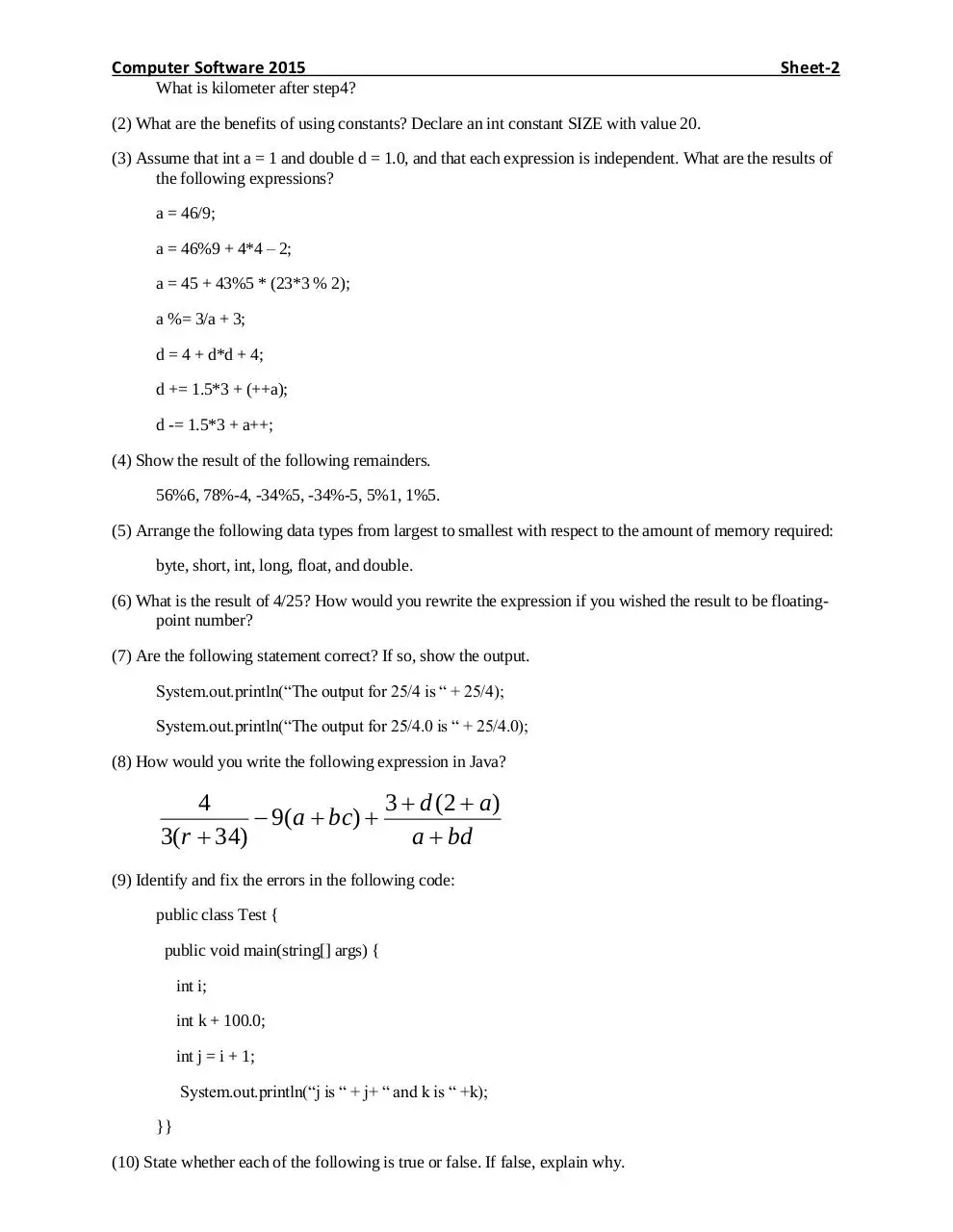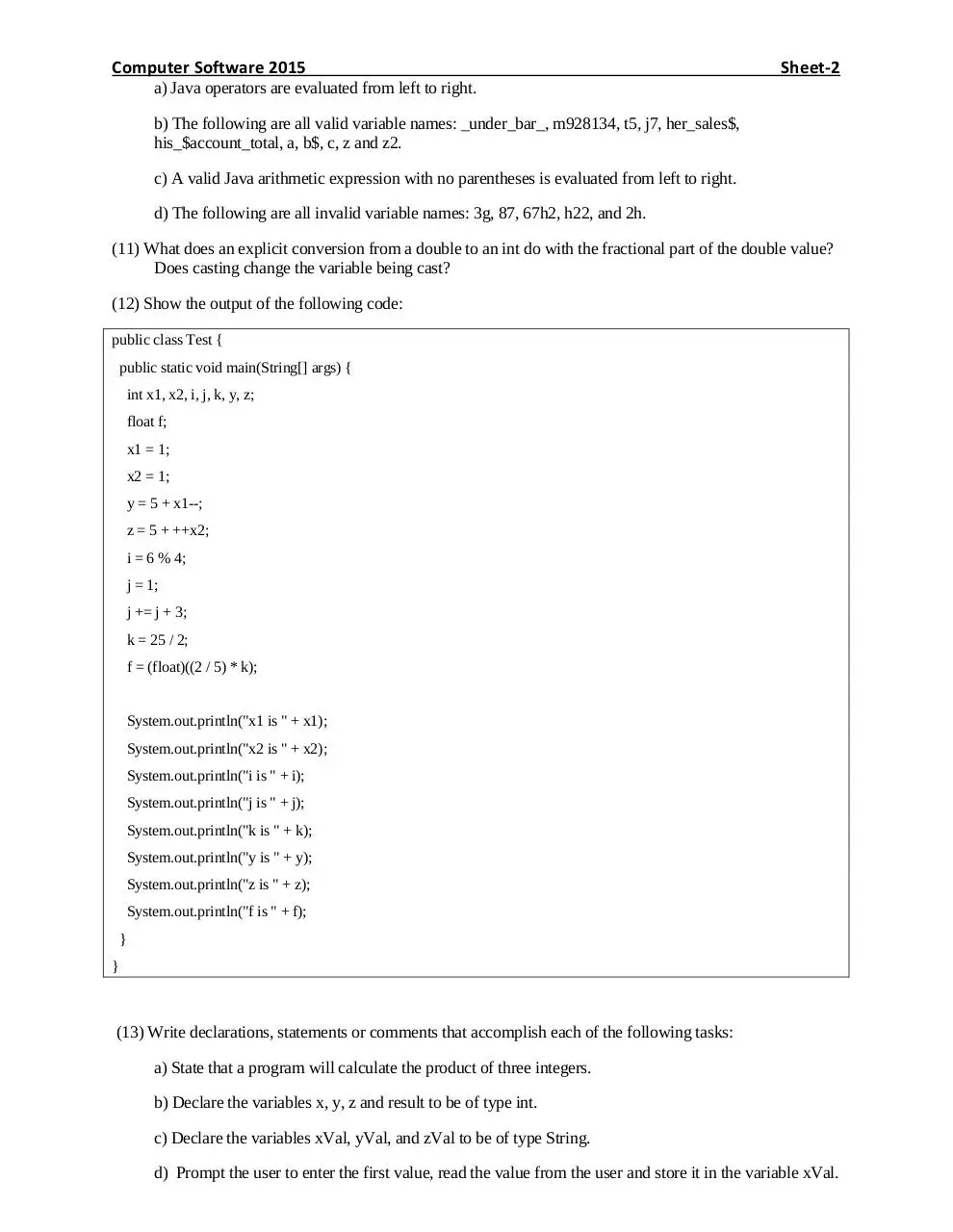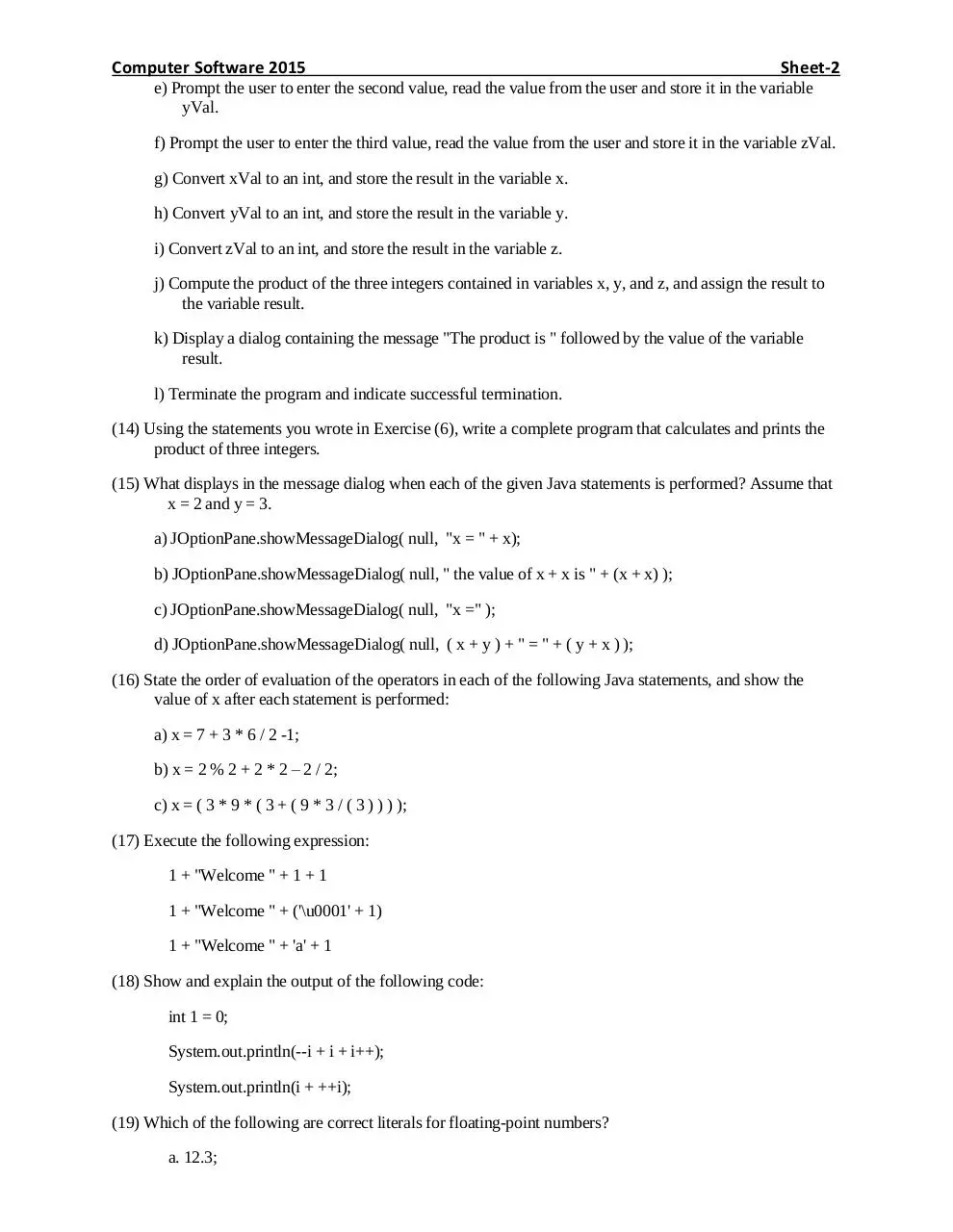Sheet-2-2015.pdf (PDF, 431.3 KB)

### Share on social networks

#### HTML Code

Copy the following HTML code to share your document on a Website or Blog